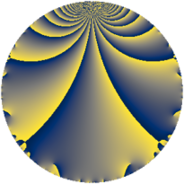# Properties

 Label 7728.2.a.bfLevel $7728$ Weight $2$ Character orbit 7728.a Self dual yes Analytic conductor $61.708$ Analytic rank $1$ Dimension $2$ CM no Inner twists $1$

# Related objects

## Newspace parameters

 Level: $$N$$ $$=$$ $$7728 = 2^{4} \cdot 3 \cdot 7 \cdot 23$$ Weight: $$k$$ $$=$$ $$2$$ Character orbit: $$[\chi]$$ $$=$$ 7728.a (trivial)

## Newform invariants

 Self dual: yes Analytic conductor: $$61.7083906820$$ Analytic rank: $$1$$ Dimension: $$2$$ Coefficient field: $$\Q(\sqrt{13})$$ Defining polynomial: $$x^{2} - x - 3$$ Coefficient ring: $$\Z[a_1, \ldots, a_{5}]$$ Coefficient ring index: $$1$$ Twist minimal: no (minimal twist has level 1932) Fricke sign: $$1$$ Sato-Tate group: $\mathrm{SU}(2)$

## $q$-expansion

Coefficients of the $$q$$-expansion are expressed in terms of $$\beta = \frac{1}{2}(1 + \sqrt{13})$$. We also show the integral $$q$$-expansion of the trace form.

 $$f(q)$$ $$=$$ $$q + q^{3} + ( -2 - \beta ) q^{5} + q^{7} + q^{9} +O(q^{10})$$ $$q + q^{3} + ( -2 - \beta ) q^{5} + q^{7} + q^{9} + ( -3 + 2 \beta ) q^{11} + ( 1 - \beta ) q^{13} + ( -2 - \beta ) q^{15} + ( -3 - 2 \beta ) q^{17} + ( 3 + 2 \beta ) q^{19} + q^{21} + q^{23} + ( 2 + 5 \beta ) q^{25} + q^{27} + ( -3 + 2 \beta ) q^{29} + ( 1 - 2 \beta ) q^{31} + ( -3 + 2 \beta ) q^{33} + ( -2 - \beta ) q^{35} + ( 3 - 2 \beta ) q^{37} + ( 1 - \beta ) q^{39} + ( 1 - 2 \beta ) q^{41} + ( -3 + \beta ) q^{43} + ( -2 - \beta ) q^{45} + ( 2 - 2 \beta ) q^{47} + q^{49} + ( -3 - 2 \beta ) q^{51} + ( 2 - 3 \beta ) q^{53} -3 \beta q^{55} + ( 3 + 2 \beta ) q^{57} + 3 \beta q^{59} + ( 1 - 3 \beta ) q^{61} + q^{63} + ( 1 + 2 \beta ) q^{65} + ( -12 + \beta ) q^{67} + q^{69} + ( -9 + 3 \beta ) q^{71} + 11 q^{73} + ( 2 + 5 \beta ) q^{75} + ( -3 + 2 \beta ) q^{77} + ( -3 + 8 \beta ) q^{79} + q^{81} + ( -11 + 4 \beta ) q^{83} + ( 12 + 9 \beta ) q^{85} + ( -3 + 2 \beta ) q^{87} + ( -9 + 5 \beta ) q^{89} + ( 1 - \beta ) q^{91} + ( 1 - 2 \beta ) q^{93} + ( -12 - 9 \beta ) q^{95} + ( 9 + 4 \beta ) q^{97} + ( -3 + 2 \beta ) q^{99} +O(q^{100})$$ $$\operatorname{Tr}(f)(q)$$ $$=$$ $$2q + 2q^{3} - 5q^{5} + 2q^{7} + 2q^{9} + O(q^{10})$$ $$2q + 2q^{3} - 5q^{5} + 2q^{7} + 2q^{9} - 4q^{11} + q^{13} - 5q^{15} - 8q^{17} + 8q^{19} + 2q^{21} + 2q^{23} + 9q^{25} + 2q^{27} - 4q^{29} - 4q^{33} - 5q^{35} + 4q^{37} + q^{39} - 5q^{43} - 5q^{45} + 2q^{47} + 2q^{49} - 8q^{51} + q^{53} - 3q^{55} + 8q^{57} + 3q^{59} - q^{61} + 2q^{63} + 4q^{65} - 23q^{67} + 2q^{69} - 15q^{71} + 22q^{73} + 9q^{75} - 4q^{77} + 2q^{79} + 2q^{81} - 18q^{83} + 33q^{85} - 4q^{87} - 13q^{89} + q^{91} - 33q^{95} + 22q^{97} - 4q^{99} + O(q^{100})$$

## Embeddings

For each embedding $$\iota_m$$ of the coefficient field, the values $$\iota_m(a_n)$$ are shown below.

For more information on an embedded modular form you can click on its label.

Label $$\iota_m(\nu)$$ $$a_{2}$$ $$a_{3}$$ $$a_{4}$$ $$a_{5}$$ $$a_{6}$$ $$a_{7}$$ $$a_{8}$$ $$a_{9}$$ $$a_{10}$$
1.1
 2.30278 −1.30278
0 1.00000 0 −4.30278 0 1.00000 0 1.00000 0
1.2 0 1.00000 0 −0.697224 0 1.00000 0 1.00000 0
 $$n$$: e.g. 2-40 or 990-1000 Significant digits: Format: Complex embeddings Normalized embeddings Satake parameters Satake angles

## Atkin-Lehner signs

$$p$$ Sign
$$2$$ $$-1$$
$$3$$ $$-1$$
$$7$$ $$-1$$
$$23$$ $$-1$$

## Inner twists

This newform does not admit any (nontrivial) inner twists.

## Twists

By twisting character orbit
Char Parity Ord Mult Type Twist Min Dim
1.a even 1 1 trivial 7728.2.a.bf 2
4.b odd 2 1 1932.2.a.c 2
12.b even 2 1 5796.2.a.m 2

By twisted newform orbit
Twist Min Dim Char Parity Ord Mult Type
1932.2.a.c 2 4.b odd 2 1
5796.2.a.m 2 12.b even 2 1
7728.2.a.bf 2 1.a even 1 1 trivial

## Hecke kernels

This newform subspace can be constructed as the intersection of the kernels of the following linear operators acting on $$S_{2}^{\mathrm{new}}(\Gamma_0(7728))$$:

 $$T_{5}^{2} + 5 T_{5} + 3$$ $$T_{11}^{2} + 4 T_{11} - 9$$ $$T_{13}^{2} - T_{13} - 3$$ $$T_{17}^{2} + 8 T_{17} + 3$$

## Hecke characteristic polynomials

$p$ $F_p(T)$
$2$ $$T^{2}$$
$3$ $$( -1 + T )^{2}$$
$5$ $$3 + 5 T + T^{2}$$
$7$ $$( -1 + T )^{2}$$
$11$ $$-9 + 4 T + T^{2}$$
$13$ $$-3 - T + T^{2}$$
$17$ $$3 + 8 T + T^{2}$$
$19$ $$3 - 8 T + T^{2}$$
$23$ $$( -1 + T )^{2}$$
$29$ $$-9 + 4 T + T^{2}$$
$31$ $$-13 + T^{2}$$
$37$ $$-9 - 4 T + T^{2}$$
$41$ $$-13 + T^{2}$$
$43$ $$3 + 5 T + T^{2}$$
$47$ $$-12 - 2 T + T^{2}$$
$53$ $$-29 - T + T^{2}$$
$59$ $$-27 - 3 T + T^{2}$$
$61$ $$-29 + T + T^{2}$$
$67$ $$129 + 23 T + T^{2}$$
$71$ $$27 + 15 T + T^{2}$$
$73$ $$( -11 + T )^{2}$$
$79$ $$-207 - 2 T + T^{2}$$
$83$ $$29 + 18 T + T^{2}$$
$89$ $$-39 + 13 T + T^{2}$$
$97$ $$69 - 22 T + T^{2}$$